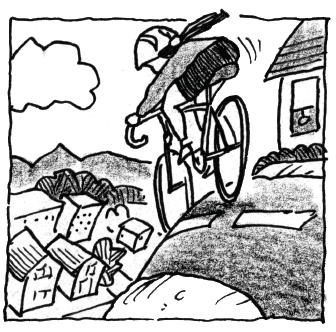### Home > APCALC > Chapter 3 > Lesson 3.2.1 > Problem3-47

3-47.

After completing the Ramp Lab in Chapter 2, Marquita decided to ride her bicycle down a nearby hill to gain more data on rolling objects. The data she collected is shown in the table below.

 Time (sec) $0.0$ $2.0$ $3.1$ $6.0$ $7.5$ $9.0$ Distance (m) $0.0$ $3.8$ $9.1$ $32.7$ $49.7$ $69.5$
1. Can Marquita use this table to determine her exact velocity at $t = 6$ Explain.

Tables only give us select values of a function. What values are we given that are close to $t = 6$? Are these values close enough to find Marquita's exact velocity at $t = 6$?

2. Approximate Marquita’s velocity at $t = 6$ using Hana’s method.

Hanna’s Method: $\frac{f(a+h)-f(a)}{h}$ where $a$ represents the value whose slope you are looking for.

Note: Since we do note know values that are infinitely close to $t = 6$, we can find Marquita's AROC (average velocity), not her IROC (actual velocity).

3. Marquita’s teacher observed that her data fits the function $d(t) = 0.789t^2 + 0.703t − 0.338$. Assuming that her teacher is correct, compute Marquita’s exact velocities at $t = 6$ and $t = 10$.

Use the Power Rule to find the IROC/derivative/slope function/velocity function. Then evaluate at $t = 6$ and again at $t = 10$.